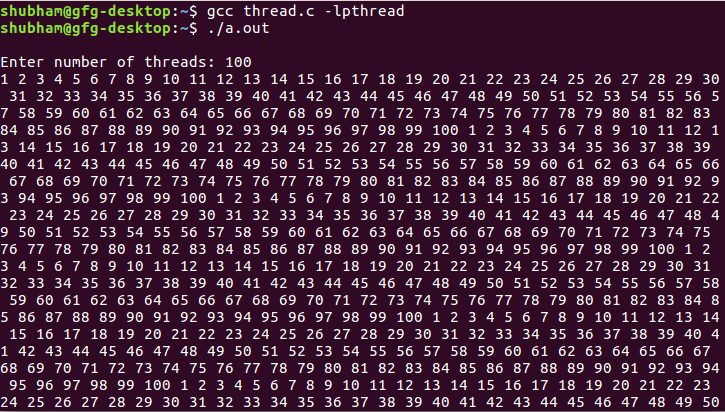# Print numbers in sequence using thread synchronization

The problem is to synchronize n number of threads using pthread library. The idea is take thread count and print 1 in first thread, print 2 in second thread, print 3 in third thread, ….. print n in nth thread and again repeat from thread 1 infinitely.

Examples :

```Input : Thread count
Output : 1 2 3 ... thread count 1 2 3 ... thread
count 1 2 3 ... thread count ....

Input : 5
Output : 1 2 3 4 5 1 2 3 4 5 1 2 3 4 5 1 2 3 4 5
1 2 3 4 5 1 2 3 4 5 ....
```

Below is the implementation:

## CPP

 `// C code to synchronize threads` `#include ` `#include ` `#include ` `#include `   `pthread_mutex_t mutex = PTHREAD_MUTEX_INITIALIZER;` `pthread_cond_t* cond = NULL;`   `int` `threads;` `volatile` `int` `cnt = 0;`   `// function to synchronize threads` `void``* foo(``void``* arg)` `{` `    ``// turn is a basically to identify a thread` `    ``int` `turn = *(``int``*)arg;`   `    ``while` `(1) {` `        ``pthread_mutex_lock(&mutex);`   `        ``// cnt is used to determne which thread should` `        ``// enter into critical section(printf() statement)` `        ``if` `(turn != cnt) {`   `            ``// put all thread except one thread in waiting` `            ``// state` `            ``pthread_cond_wait(&cond[turn], &mutex);` `        ``}`   `        ``// it's a time to print turn can have` `        ``// values starting from 0. Hence + 1` `        ``printf``(``"%d "``, turn + 1);`   `        ``// detemine which thread need to be scheduled now` `        ``if` `(cnt < threads - 1) {` `            ``cnt++;` `        ``}` `        ``else` `{` `            ``cnt = 0;` `        ``}`   `        ``// weak up next thread` `        ``pthread_cond_signal(&cond[cnt]);` `        ``pthread_mutex_unlock(&mutex);` `    ``}`   `    ``return` `NULL;` `}`   `// Driver code` `int` `main()` `{` `    ``pthread_t* tid;` `    ``volatile` `int` `i;` `    ``int``* arr;`   `    ``printf``(``"\nEnter number of threads: "``);` `    ``scanf``(``"%d"``, &threads);`   `    ``// allocate memory to cond (conditional variable),` `    ``// thread id's and array of size threads` `    ``cond = (pthread_cond_t*)``malloc``(``sizeof``(pthread_cond_t)` `                                   ``* threads);` `    ``tid = (pthread_t*)``malloc``(``sizeof``(pthread_t) * threads);` `    ``arr = (``int``*)``malloc``(``sizeof``(``int``) * threads);`   `    ``// Initialize cond (conditional variable)` `    ``for` `(``int` `i = 0; i < threads; i++) {` `        ``if` `(pthread_cond_init(&cond[i], NULL) != 0) {` `            ``perror``(``"pthread_cond_init() error"``);` `            ``exit``(1);` `        ``}` `    ``}`   `    ``// create threads` `    ``for` `(i = 0; i < threads; i++) {` `        ``arr[i] = i;` `        ``pthread_create(&tid[i], NULL, foo, (``void``*)&arr[i]);` `    ``}`   `    ``// waiting for thread` `    ``for` `(i = 0; i < threads; i++) {` `        ``pthread_join(tid[i], NULL);` `    ``}`   `    ``return` `0;` `}`

Output :Attention reader! Don’t stop learning now. Get hold of all the important DSA concepts with the DSA Self Paced Course at a student-friendly price and become industry ready.

My Personal Notes arrow_drop_upHello I am Sacheen Birhade from Pune I have 7+ years of experience in enterprise Software Development I holds two master degrees viz MCA and MSc Computer Science I am enthusiastic about programming data structure Linux System Programming and Multithreading You can reach me at spbirhade[at]gmail[dot

If you like GeeksforGeeks and would like to contribute, you can also write an article using contribute.geeksforgeeks.org or mail your article to contribute@geeksforgeeks.org. See your article appearing on the GeeksforGeeks main page and help other Geeks.

Please Improve this article if you find anything incorrect by clicking on the "Improve Article" button below.

Improved By : jeff1jeffo

Article Tags :
Practice Tags :

1

Please write to us at contribute@geeksforgeeks.org to report any issue with the above content.